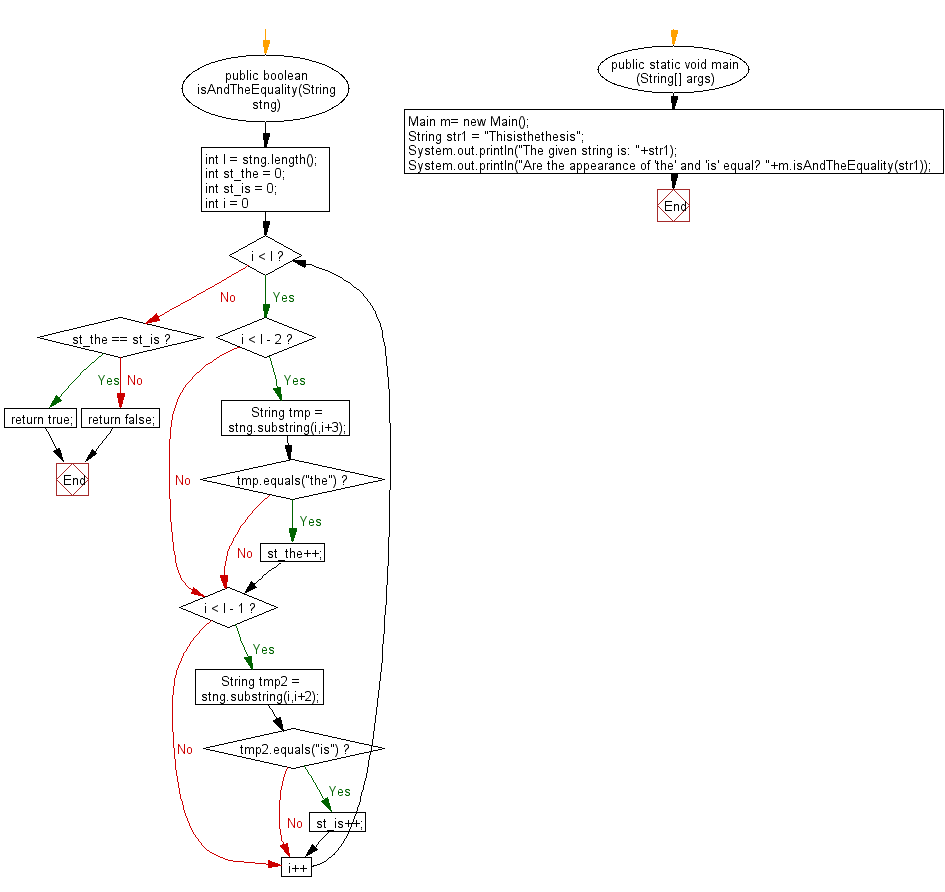﻿ Java - The number of times 2 substrings appear in the string

# Java: Check the number of appearances of the two substrings appear anywhere in the string

## Java String: Exercise-90 with Solution

Write a Java program to check the number of times the two substrings appearing anywhere in a string.

Visual Presentation:Sample Solution:

Java Code:

``````import java.util.*;

// Define a class named Main
public class Main {

// Method to check the equality of appearances of "the" and "is" in the string
public boolean isAndTheEquality(String stng) {
int l = stng.length(); // Get the length of the given string
int st_the = 0; // Initialize a counter for occurrences of "the"
int st_is = 0; // Initialize a counter for occurrences of "is"

// Loop through the string to find occurrences of "the" and "is"
for (int i = 0; i < l; i++) {
// Check for occurrences of "the" (a three-character substring)
if (i < l - 2) {
String tmp = stng.substring(i, i + 3);
if (tmp.equals("the"))
st_the++;
}
// Check for occurrences of "is" (a two-character substring)
if (i < l - 1) {
String tmp2 = stng.substring(i, i + 2);
if (tmp2.equals("is"))
st_is++;
}
}

// Check if the counts of "the" and "is" occurrences are equal
if (st_the == st_is)
return true;
else
return false;
}

// Main method to execute the program
public static void main(String[] args) {
Main m = new Main(); // Create an instance of the Main class

String str1 = "Thisisthethesis"; // Given input string

// Display the given string and whether the appearances of "the" and "is" are equal
System.out.println("The given string is: " + str1);
System.out.println("Are the appearances of 'the' and 'is' equal? " + m.isAndTheEquality(str1));
}
}
``````

Sample Output:

```The given string is: Thisisthethesis
Are the appearance of 'the' and 'is' equal? false
```

Flowchart:Java Code Editor:

Improve this sample solution and post your code through Disqus

What is the difficulty level of this exercise?

Test your Programming skills with w3resource's quiz.

﻿